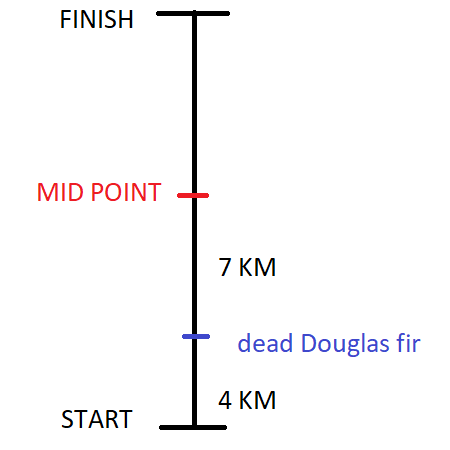# At the beginning of a run on a road that goes due north, Hayden noticed that she was 4 kilometers...

## Question:

At the beginning of a run on a road that goes due north, Hayden noticed that she was {eq}4 {/eq} kilometers south of a dead Douglas fir. If the midpoint of her jog was {eq}7 {/eq} kilometers north of the dead Douglas fir, how far did Hayden have to travel?

## Algebraic word problem

All word problem is a simplified way to express the relationship between variables and constants that makes it easier to understand and communicate.

To solve a word problem we need to understand the expression, assign unknown variables and make a valid equation out of it.

For example, Three-pound of fish and some chicken made the bag weight 5 pounds. In this statement, the constants are the weight of fish and total weight and the unknown variable is the weight of the chicken. We can make the following observation that the combined weight of fish and chicken is 5 pounds.

$$\text{Weight of fish + Weight of chicken = Total weight}$$

Substituting value will give us the unknown variable.

$$\text{3+ Weight of chicken = 5}$$

$$\text{ Weight of chicken = 2\ lb}$$

Hayden starts from a point {eq}4\ Km {/eq} south of the dead Douglas fir and the midpoint is {eq}7\ km {/eq}north of the dead Douglas fir. The scenario can easily be understood by the image below.The midpoint of Hayden's run is the sum of distance traveled from starting point to the dead Douglas fir and from dead Douglas fir to the midpoint.

$$\text{Start to Midpoint} =4\ km + 7\ km$$

$$\text{Start to Midpoint} = 11\ km$$

Total distance is twice the distance between start to midpoint.

$$\text{Total distance}= 2\times \text{Start to Midpoint}$$

$$\text{Total distance}= 2\times 11$$

$$\text{Total distance}= 22\ km$$

Total distance run by Hayden is {eq}22\ Km. {/eq}